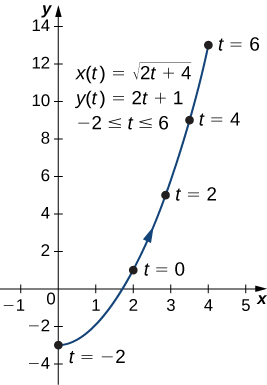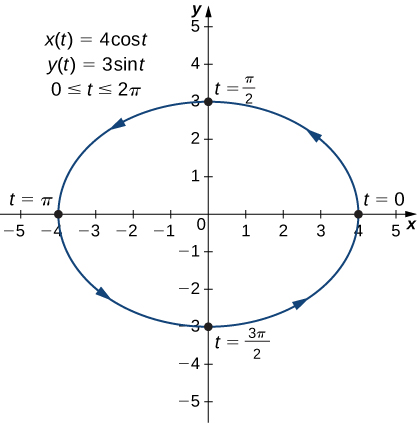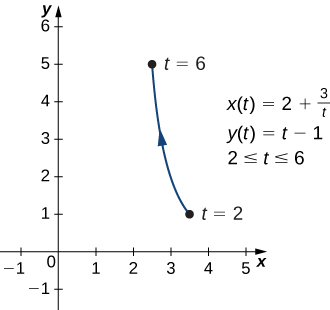# 1.1 Parametric equations  (Page 3/14)

 Page 3 / 14

## Eliminating the parameter

Eliminate the parameter for each of the plane curves described by the following parametric equations and describe the resulting graph.

1. $x\left(t\right)=\sqrt{2t+4},\phantom{\rule{1em}{0ex}}y\left(t\right)=2t+1,\phantom{\rule{1em}{0ex}}-2\le t\le 6$
2. $x\left(t\right)=4\phantom{\rule{0.2em}{0ex}}\text{cos}\phantom{\rule{0.2em}{0ex}}t,\phantom{\rule{1em}{0ex}}y\left(t\right)=3\phantom{\rule{0.2em}{0ex}}\text{sin}\phantom{\rule{0.2em}{0ex}}t,\phantom{\rule{1em}{0ex}}0\le t\le 2\pi$
1. To eliminate the parameter, we can solve either of the equations for t. For example, solving the first equation for t gives
$\begin{array}{ccc}\hfill x& =\hfill & \sqrt{2t+4}\hfill \\ \hfill {x}^{2}& =\hfill & 2t+4\hfill \\ \hfill {x}^{2}-4& =\hfill & 2t\hfill \\ \hfill t& =\hfill & \frac{{x}^{2}-4}{2}.\hfill \end{array}$

Note that when we square both sides it is important to observe that $x\ge 0.$ Substituting $t=\frac{{x}^{2}-4}{2}$ this into $y\left(t\right)$ yields
$\begin{array}{ccc}\hfill y\left(t\right)& =\hfill & 2t+1\hfill \\ \hfill y& =\hfill & 2\left(\frac{{x}^{2}-4}{2}\right)+1\hfill \\ \hfill y& =\hfill & {x}^{2}-4+1\hfill \\ \hfill y& =\hfill & {x}^{2}-3.\hfill \end{array}$

This is the equation of a parabola opening upward. There is, however, a domain restriction because of the limits on the parameter t . When $t=-2,$ $x=\sqrt{2\left(-2\right)+4}=0,$ and when $t=6,$ $x=\sqrt{2\left(6\right)+4}=4.$ The graph of this plane curve follows.Graph of the plane curve described by the parametric equations in part a.
2. Sometimes it is necessary to be a bit creative in eliminating the parameter. The parametric equations for this example are
$x\left(t\right)=4\phantom{\rule{0.2em}{0ex}}\text{cos}\phantom{\rule{0.2em}{0ex}}t\phantom{\rule{0.2em}{0ex}}\text{and}\phantom{\rule{0.2em}{0ex}}y\left(t\right)=3\phantom{\rule{0.2em}{0ex}}\text{sin}\phantom{\rule{0.2em}{0ex}}t.$

Solving either equation for t directly is not advisable because sine and cosine are not one-to-one functions. However, dividing the first equation by 4 and the second equation by 3 (and suppressing the t ) gives us
$\text{cos}\phantom{\rule{0.2em}{0ex}}t=\frac{x}{4}\phantom{\rule{0.2em}{0ex}}\text{and}\phantom{\rule{0.2em}{0ex}}\text{sin}\phantom{\rule{0.2em}{0ex}}t=\frac{y}{3}.$

Now use the Pythagorean identity ${\text{cos}}^{2}t+{\text{sin}}^{2}t=1$ and replace the expressions for $\text{sin}\phantom{\rule{0.2em}{0ex}}t$ and $\text{cos}\phantom{\rule{0.2em}{0ex}}t$ with the equivalent expressions in terms of x and y . This gives
$\begin{array}{ccc}\hfill {\left(\frac{x}{4}\right)}^{2}+{\left(\frac{y}{3}\right)}^{2}& =\hfill & 1\hfill \\ \hfill \frac{{x}^{2}}{16}+\frac{{y}^{2}}{9}& =\hfill & 1.\hfill \end{array}$

This is the equation of a horizontal ellipse centered at the origin, with semimajor axis 4 and semiminor axis 3 as shown in the following graph.Graph of the plane curve described by the parametric equations in part b.
As t progresses from $0$ to $2\pi ,$ a point on the curve traverses the ellipse once, in a counterclockwise direction. Recall from the section opener that the orbit of Earth around the Sun is also elliptical. This is a perfect example of using parameterized curves to model a real-world phenomenon.

Eliminate the parameter for the plane curve defined by the following parametric equations and describe the resulting graph.

$x\left(t\right)=2+\frac{3}{t},\phantom{\rule{1em}{0ex}}y\left(t\right)=t-1,\phantom{\rule{1em}{0ex}}2\le t\le 6$

$x=2+\frac{3}{y+1},$ or $y=-1+\frac{3}{x-2}.$ This equation describes a portion of a rectangular hyperbola centered at $\left(2,-1\right).$So far we have seen the method of eliminating the parameter, assuming we know a set of parametric equations that describe a plane curve. What if we would like to start with the equation of a curve and determine a pair of parametric equations for that curve? This is certainly possible, and in fact it is possible to do so in many different ways for a given curve. The process is known as parameterization of a curve    .

## Parameterizing a curve

Find two different pairs of parametric equations to represent the graph of $y=2{x}^{2}-3.$

First, it is always possible to parameterize a curve by defining $x\left(t\right)=t,$ then replacing x with t in the equation for $y\left(t\right).$ This gives the parameterization

$x\left(t\right)=t,\phantom{\rule{1em}{0ex}}y\left(t\right)=2{t}^{2}-3.$

Since there is no restriction on the domain in the original graph, there is no restriction on the values of t.

We have complete freedom in the choice for the second parameterization. For example, we can choose $x\left(t\right)=3t-2.$ The only thing we need to check is that there are no restrictions imposed on x ; that is, the range of $x\left(t\right)$ is all real numbers. This is the case for $x\left(t\right)=3t-2.$ Now since $y=2{x}^{2}-3,$ we can substitute $x\left(t\right)=3t-2$ for x. This gives

$\begin{array}{cc}\hfill y\left(t\right)& =2{\left(3t-2\right)}^{2}-2\hfill \\ & =2\left(9{t}^{2}-12t+4\right)-2\hfill \\ & =18{t}^{2}-24t+8-2\hfill \\ & =18{t}^{2}-24t+6.\hfill \end{array}$

Therefore, a second parameterization of the curve can be written as

$x\left(t\right)=3t-2\phantom{\rule{0.2em}{0ex}}\text{and}\phantom{\rule{0.2em}{0ex}}y\left(t\right)=18{t}^{2}-24t+6.$

where we get a research paper on Nano chemistry....?
nanopartical of organic/inorganic / physical chemistry , pdf / thesis / review
Ali
what are the products of Nano chemistry?
There are lots of products of nano chemistry... Like nano coatings.....carbon fiber.. And lots of others..
learn
Even nanotechnology is pretty much all about chemistry... Its the chemistry on quantum or atomic level
learn
da
no nanotechnology is also a part of physics and maths it requires angle formulas and some pressure regarding concepts
Bhagvanji
hey
Giriraj
Preparation and Applications of Nanomaterial for Drug Delivery
revolt
da
Application of nanotechnology in medicine
what is variations in raman spectra for nanomaterials
I only see partial conversation and what's the question here!
what about nanotechnology for water purification
please someone correct me if I'm wrong but I think one can use nanoparticles, specially silver nanoparticles for water treatment.
Damian
yes that's correct
Professor
I think
Professor
Nasa has use it in the 60's, copper as water purification in the moon travel.
Alexandre
nanocopper obvius
Alexandre
what is the stm
is there industrial application of fullrenes. What is the method to prepare fullrene on large scale.?
Rafiq
industrial application...? mmm I think on the medical side as drug carrier, but you should go deeper on your research, I may be wrong
Damian
How we are making nano material?
what is a peer
What is meant by 'nano scale'?
What is STMs full form?
LITNING
scanning tunneling microscope
Sahil
how nano science is used for hydrophobicity
Santosh
Do u think that Graphene and Fullrene fiber can be used to make Air Plane body structure the lightest and strongest. Rafiq
Rafiq
what is differents between GO and RGO?
Mahi
what is simplest way to understand the applications of nano robots used to detect the cancer affected cell of human body.? How this robot is carried to required site of body cell.? what will be the carrier material and how can be detected that correct delivery of drug is done Rafiq
Rafiq
if virus is killing to make ARTIFICIAL DNA OF GRAPHENE FOR KILLED THE VIRUS .THIS IS OUR ASSUMPTION
Anam
analytical skills graphene is prepared to kill any type viruses .
Anam
Any one who tell me about Preparation and application of Nanomaterial for drug Delivery
Hafiz
what is Nano technology ?
write examples of Nano molecule?
Bob
The nanotechnology is as new science, to scale nanometric
brayan
nanotechnology is the study, desing, synthesis, manipulation and application of materials and functional systems through control of matter at nanoscale
Damian
Is there any normative that regulates the use of silver nanoparticles?
what king of growth are you checking .?
Renato
What fields keep nano created devices from performing or assimulating ? Magnetic fields ? Are do they assimilate ?
why we need to study biomolecules, molecular biology in nanotechnology?
?
Kyle
yes I'm doing my masters in nanotechnology, we are being studying all these domains as well..
why?
what school?
Kyle
biomolecules are e building blocks of every organics and inorganic materials.
Joe
can you provide the details of the parametric equations for the lines that defince doubly-ruled surfeces (huperbolids of one sheet and hyperbolic paraboloid). Can you explain each of the variables in the equations?By JavaChamp TeamByBy Monty HartfieldBy OpenStaxBy Steve GibbsBy Robert MurphyBy Vongkol HENGBy Richley CrapoBy OpenStaxBy Eddie Unverzagt